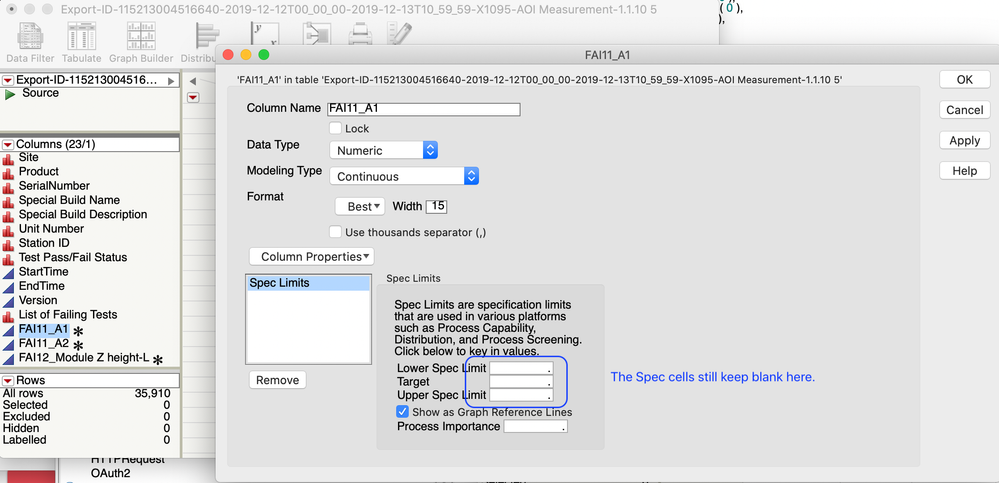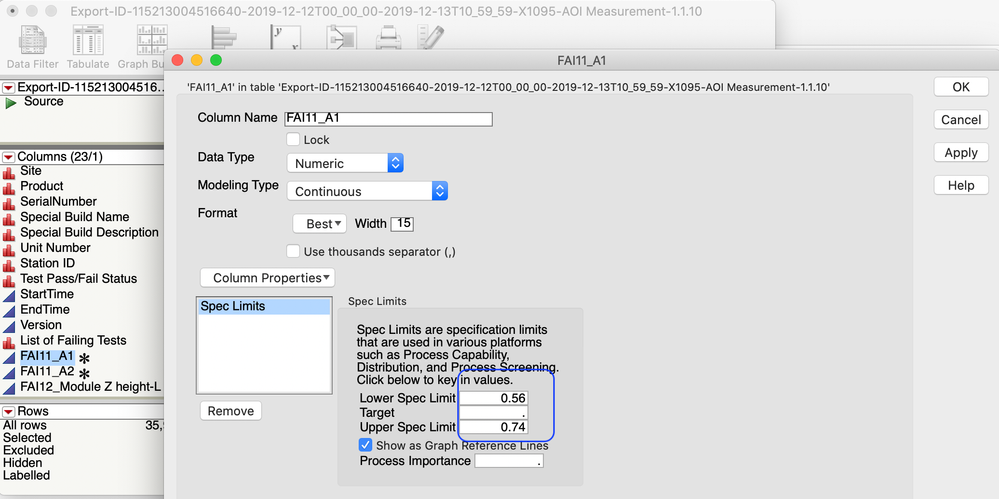Choose Language Hide Translation Bar
Highlighted

## Set Spec Limits of columns with column stored value

Hi All,

I imported csv files into JMP to get data table (assigned as dt0) and spec table (assigned as dt1) respectively. I wonder to get the spec in spec table (dt0) and set Spec Limits of columns in data table (dt0).

The issue I found that the specs can not be set successfully:Here are my codes:

Names Default To Here( 1 );

Files = Pick File(

"Select CSV File",

{"JMP Files|jmp;jsl;jrn", "All Files|*"},

1,

0,

""

);

dt0 = Open(

Files,

Import Settings(

End Of Line( CRLF, CR, LF ),

End Of Field( Comma, CSV( 0 ) ),

Strip Quotes( 1 ),

Use Apostrophe as Quotation Mark( 0 ),

Use Regional Settings( 0 ),

Scan Whole File( 1 ),

Treat empty columns as numeric( 0 ),

CompressNumericColumns( 0 ),

CompressCharacterColumns( 0 ),

CompressAllowListCheck( 0 ),

Labels( 1 ),

Column Names Start( 2 ),

Data Starts( 8 ),

Year Rule( "20xx" )

)

);

dt1 = Open(

Files,

Import Settings(

End Of Line( CRLF, CR, LF ),

End Of Field( Comma, CSV( 0 ) ),

Strip Quotes( 1 ),

Use Apostrophe as Quotation Mark( 0 ),

Use Regional Settings( 0 ),

Scan Whole File( 1 ),

Treat empty columns as numeric( 0 ),

CompressNumericColumns( 0 ),

CompressCharacterColumns( 0 ),

CompressAllowListCheck( 0 ),

Labels( 1 ),

Column Names Start( 2 ),

Data Starts( 5 ),

Year Rule( "20xx" )

)

);

For(i=13, i<=N Cols(dt0), i++,

Column(dt0,i)<<Data Type(Numeric);

Column(dt0,i)<<Modeling Type("Continuous");

);

For(i=13, i<=N Cols(dt1), i++,

Column(dt1,i)<<Data Type(Numeric);

Column(dt1,i)<<Modeling Type("Continuous");

);

For(i=13, i<=N Cols(dt1), i++,

tempUSL=Col Stored Value(dt1, i, 1);           //Assign the spec from dt1 spec table to 'tempUSL' and 'tempLSL'

tempLSL=Col Stored Value(dt1, i, 2);

Eval(

Eval Expr(

Column( dt0,i ) << Set Property(

"Spec Limits",

{LSL( Expr( tempLSL ) ), USL( Expr( tempUSL ) ), Show Limits( 1 )}

)

)

);

);

1 ACCEPTED SOLUTION

Accepted Solutions
Highlighted

## Re: Set Spec Limits of columns with column stored value

Hi All,

I found the solution by using these codes:

``````Eval(
Eval Expr(
tempUSL=Col Stored Value(dt1, Expr(i), 1)
)
);
Eval(
Eval Expr(
tempLSL=Col Stored Value(dt1, Expr(i), 2)
)
);``````

Thanks

4 REPLIES 4
Highlighted

## Re: Set Spec Limits of columns with column stored value

If assign a number to 'tempUSL' and 'tempLSL' directly, it could work.

But I need to assign the spec limits from spec table.

``````Names Default To Here( 1 );
Files = Pick File(
"Select CSV File",
{"JMP Files|jmp;jsl;jrn", "All Files|*"},
1,
0,
""
);

dt0 = Open(
Files,
Import Settings(
End Of Line( CRLF, CR, LF ),
End Of Field( Comma, CSV( 0 ) ),
Strip Quotes( 1 ),
Use Apostrophe as Quotation Mark( 0 ),
Use Regional Settings( 0 ),
Scan Whole File( 1 ),
Treat empty columns as numeric( 0 ),
CompressNumericColumns( 0 ),
CompressCharacterColumns( 0 ),
CompressAllowListCheck( 0 ),
Labels( 1 ),
Column Names Start( 2 ),
Data Starts( 8 ),
Year Rule( "20xx" )
)
);

dt1 = Open(
Files,
Import Settings(
End Of Line( CRLF, CR, LF ),
End Of Field( Comma, CSV( 0 ) ),
Strip Quotes( 1 ),
Use Apostrophe as Quotation Mark( 0 ),
Use Regional Settings( 0 ),
Scan Whole File( 1 ),
Treat empty columns as numeric( 0 ),
CompressNumericColumns( 0 ),
CompressCharacterColumns( 0 ),
CompressAllowListCheck( 0 ),
Labels( 1 ),
Column Names Start( 2 ),
Data Starts( 5 ),
Year Rule( "20xx" )
)
);

For(i=13, i<=N Cols(dt0), i++,
Column(dt0,i)<<Data Type(Numeric);
Column(dt0,i)<<Modeling Type("Continuous");
);

For(i=13, i<=N Cols(dt1), i++,
Column(dt1,i)<<Data Type(Numeric);
Column(dt1,i)<<Modeling Type("Continuous");
);

For(i=13, i<=N Cols(dt1), i++,
tempUSL=0.74;
tempLSL=0.56;
tempTarget=(tempUSL+tempLSL)/2;
Eval(
Eval Expr(
Column( dt0,i ) << Set Property(
"Spec Limits",
{LSL( Expr( tempLSL ) ), USL( Expr( tempUSL ) ), Show Limits( 1 )}
)
)
);
);``````Highlighted

## Re: Set Spec Limits of columns with column stored value

Hi All,

I found the solution by using these codes:

``````Eval(
Eval Expr(
tempUSL=Col Stored Value(dt1, Expr(i), 1)
)
);
Eval(
Eval Expr(
tempLSL=Col Stored Value(dt1, Expr(i), 2)
)
);``````

Thanks

Highlighted

## Re: Set Spec Limits of columns with column stored value

I see what you're doing and I have to say your code looks very nice. Also very complex.

There are several other ways to get the same thing accomplished by scripting platforms that already exist in JMP.

Here are are a couple of examples:

This one uses the process screening platfom

``````Names Default To Here( 1 );
dt1 = Open( "\$SAMPLE_DATA/Cities.jmp" );
dt2 = Open( "\$SAMPLE_DATA/CitySpecLimits.jmp" );
dt1 << Process Screening(
Y( :OZONE, :CO, :SO2, :NO ),
Use Limits Table(
1,
dt2,
Process Variables( :Column 1 ),
LSL( :_LSL ),
USL( :_USL ),
Target( :_Target ),
Go
)
);``````

This one uses the process capability platform

``````Names Default To Here( 1 );
dt = Open( "\$SAMPLE_DATA/Cities.jmp" );
obj = dt << Process Capability(
Process Variables( :OZONE, :CO, :SO2, :NO ),
Spec Limits(
Import Spec Limits(
"\$SAMPLE_DATA/CitySpecLimits.jmp"
)
)
);``````

In this example, a dialog opens to allow the user to select the spec limit table

``````Names Default To Here( 1 );
dt = Open( "\$SAMPLE_DATA/Semiconductor Capability.jmp" );
obj = dt << Manage Spec Limits(
Y( dt << Get Column Group( "Processes" ) )
);``````

This basics code which takes advantage of platform features, is much easier to maintain too.

Hope this was useful,

Cheers,

B

JMP Systems Engineer, Pharm and BioPharm Sciences
Highlighted

## Re: Set Spec Limits of columns with column stored value

Very good advice, it really helps. Thank you very much, Byron!

Article Labels

There are no labels assigned to this post.